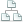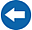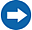Home >Tips >Excel 2016 from Scratch >Relative cell referencesSite map

# Excel 2016 from Scratch Exercise Relative Cell Reference

## An Excel tutorial by Peter KalmstromIn this demo in the Excel 2016 from Scratch series Peter Kalmstrom explains how formulas with relative cell references work.

Peter calculates Buy values, Current values and Differences of Stock by using formulas for plus, minus and multiplication.

### Exercise

If you want to try the exercise yourself, please download the Excel file Peter uses in the demo!

### Content

This is what Peter shows in the demo below:

• How to add a currency to cells with numbers.
• How to start a calculation.
• What a relative reference means.
• How to create a formula that multiplies two cells with relative references.
• How to create a formula that calculates the difference between two cells with relative references.
• How to calculate the sum of a column using the AutoSum button.
• How to expand formulas with relative references to more cells.
Peter uses Excel 2016 for his demo, but the Excel basics are the same for earlier versions of Excel.